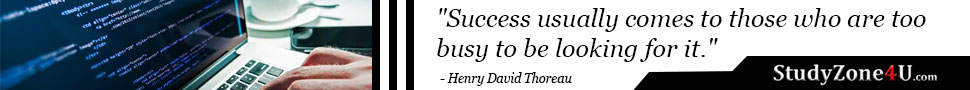# What will be the output of \$a and \$b once code bellow is executed?

```\$a = '1';
\$b = &\$a;
\$b = "2\$b";```

Last updated 4 years ago | 982 views

Tags:- PHP# PHP | output of \$a and \$b once this code \$a = '1'; \$b = &\$a; \$b = "2\$b" executed;

Both \$a and \$b will output a string "21".

Because the statement \$b = &\$a; sets \$b equal to a reference to \$a. Thereafter, as long as \$b remains a reference to \$a, anything done to \$a will affect \$b and vice versa.

So when the statement \$b = "2\$b" executes, \$b is set equals to string "2" followed by the current value of \$b which is 1, so this results of \$b sets to string "21" (i.e., the concatenation of "2" and "1"). And, since \$b is a reference to \$a, so any things change to anyone will affect both, therefore both \$a and \$b will output the string "21".